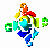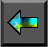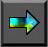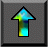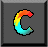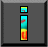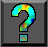Next: 1 Parallel Computers and Computation Up: Part I: Concepts Previous: Part I: Concepts

# Part I: Concepts

The first part of this book comprises four chapters that deal with the design of parallel programs. Chapter 1 briefly introduces parallel computation and the importance of concurrency, scalability, locality, and modularity in parallel algorithm design. It also introduces the machine model and programming model used when developing parallel algorithms in subsequent chapters.

Chapter 2 presents a design methodology for parallel programs whereby the design process is divided into distinct partition, communication, agglomeration, and mapping phases. It introduces commonly used techniques and shows how these apply to realistic problems. It also presents design checklists for each phase. Case studies illustrate the application of the design methodology.

Chapter 3 describes how to analyze the performance of parallel algorithms and parallel programs, and shows how simple performance models can be used to assist in the evaluation of both design alternatives and program implementations. It also shows how performance models can account for characteristics of realistic interconnection networks.

Finally, Chapter 4 considers the issues that arise when parallel algorithms are combined to develop larger programs. It reviews the basic principles of modular design and discusses how these apply to parallel programs. It also examines different approaches to the composition of parallel program modules and shows how to model the performance of multicomponent programs.Next: 1 Parallel Computers and Computation Up: Part I: Concepts Previous: Part I: Concepts